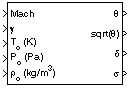# Relative Ratio

Calculate relative atmospheric ratios

• Library:
• Aerospace Blockset / Flight Parameters

•## Description

The Relative Ratio block computes the relative atmospheric ratios, including the relative temperature ratio (θ), $\sqrt{\theta }$, relative pressure ratio (δ), and relative density ratio (σ).

θ represents the ratio of the air stream temperature at a chosen reference station relative to sea level standard atmospheric conditions:

`$\theta =\frac{T}{{T}_{0}}.$`

δ represents the ratio of the air stream pressure at a chosen reference station relative to sea level standard atmospheric conditions:

`$\delta =\frac{P}{{P}_{0}}.$`

σ represents the ratio of the air stream density at a chosen reference station relative to sea level standard atmospheric conditions:

`$\sigma =\frac{\rho }{{\rho }_{0}}.$`

The Relative Ratio block icon displays the input units selected from the Units parameter.

## Limitations

For cases in which total temperature, total pressure, or total density ratio is desired (Mach number is nonzero), the total temperature, total pressure, and total densities are calculated assuming perfect gas (with constant molecular weight, constant pressure specific heat, and constant specific heat ratio) and dry air.

## Ports

### Input

expand all

Mach number, specified as a scalar.

Data Types: `double`

Ratio between the specific heat at constant pressure (Cp) and the specific heat at constant volume (Cv), specified as a scalar. For example, (γ = Cp/Cv).

Data Types: `double`

Static temperature, specified as a scalar.

Data Types: `double`

Static pressure, specified as a scalar.

Data Types: `double`

Static density, specified as a scalar.

Data Types: `double`

### Output

expand all

Relative temperature ratio (θ), returned as a scalar.

#### Dependencies

To enable this port, select Theta.

Data Types: `double`

Square root of the relative temperature ratio ( $\sqrt{\theta }$), returned as a scalar.

#### Dependencies

To enable this port, select Square root of theta.

Data Types: `double`

Relative pressure ratio, (δ), returned as a scalar.

#### Dependencies

To enable this port, select Delta.

Data Types: `double`

Relative density ratio, (σ), returned as a scalar.

#### Dependencies

To enable this port, select Sigma.

Data Types: `double`

## Parameters

expand all

Input units, specified as:

UnitsTstaticPstaticrho_static
`Metric (MKS)` KelvinPascalKilograms per cubic meter
`English` Degrees RankinePound force per square inchSlug per cubic foot

#### Programmatic Use

 Block Parameter: `units` Type: character vector Values: ```'Metric (MKS)'``` | `'English'` Default: ```'Metric (MKS)'```

When selected, the block calculates the relative temperature ratio (θ) and static temperature is a required input.

#### Programmatic Use

 Block Parameter: `theta` Type: character vector Values: `'on'` | `'off'` Default: `'on'`

When selected, the block calculates the square root of relative temperature ratio ( $\sqrt{\theta }$) and static temperature is a required input.

#### Dependencies

Selecting this check box enables the sqrt(θ) output port.

#### Programmatic Use

 Block Parameter: `sq_theta` Type: character vector Values: `'on'` | `'off'` Default: `'on'`

When selected, the block calculates the relative pressure ratio (δ) and static pressure is a required input.

#### Programmatic Use

 Block Parameter: `delta` Type: character vector Values: `'on'` | `'off'` Default: `'on'`

When selected, the block the relative density ratio (σ) and static density is a required input.

#### Programmatic Use

 Block Parameter: `sigma` Type: character vector Values: `'on'` | `'off'` Default: `'on'`

 Aeronautical Vestpocket Handbook, United Technologies Pratt & Whitney, August, 1986.

##### SupportGet trial now